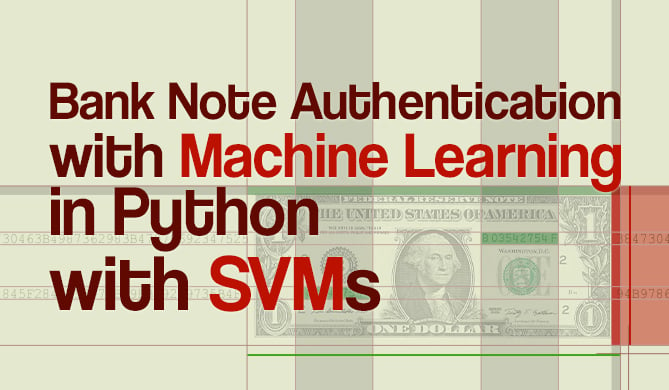How to Save and Load Scikit-Learn Model

# How to Save and Load Scikit-Learn ModelSaving and loading Scikit-Learn models is part of the lifecycle of most models - typically, you'll train them in one runtime and serve them in another.

In this Byte - you'll learn how to save and load a regressor using Scikit-Learn. First off, let's build a simple regressor and fit it:

from sklearn import datasets
from sklearn.model_selection import train_test_split
from sklearn.preprocessing import MinMaxScaler
from sklearn import ensemble

X, y = datasets.fetch_california_housing(return_X_y=True)
X_train, X_test, y_train, y_test = train_test_split(X, y)

scaler = MinMaxScaler()
X_train_scaled = scaler.fit_transform(X_train)
X_test_scaled = scaler.transform(X_test)

model = ensemble.RandomForestRegressor().fit(X_train_scaled, y_train)


With the model fit - let's go ahead and save it.Note: The data is scaled for the model to learn from. You'll want to save the state of this scaler as well, and load it to preprocess the data when the model is used for inference. To learn more about saving scalers - read "How to Save and Load Fit Scikit-Learn Scalers"!

## Pickle

pickle is a generally popular and widely-used serialization package, that can easily store and retrieve Scikit-Learn models:

Get free courses, guided projects, and more

import pickle

print('Model score:', model.score(X_test_scaled, y_test))
pickle.dump(model, open('rfr_model.sav', 'wb'))



This results in:

Model score: 0.7974923279678516


## Joblib

Generally speaking - joblib is faster with larger arrays, however, for loading and saving models, it makes no practical difference:

import joblib

print('Model score:', model.score(X_test_scaled, y_test))
joblib.dump(model, 'rfr_model.sav')



This results in:

Model score: 0.7974923279678516

Last Updated: July 1st, 2022
David LandupAuthor

Entrepreneur, Software and Machine Learning Engineer, with a deep fascination towards the application of Computation and Deep Learning in Life Sciences (Bioinformatics, Drug Discovery, Genomics), Neuroscience (Computational Neuroscience), robotics and BCIs.

Great passion for accessible education and promotion of reason, science, humanism, and progress.Project

### Bank Note Fraud Detection with SVMs in Python with Scikit-Learn

# python# machine learning# scikit-learn# data science

Can you tell the difference between a real and a fraud bank note? Probably! Can you do it for 1000 bank notes? Probably! But it...Details